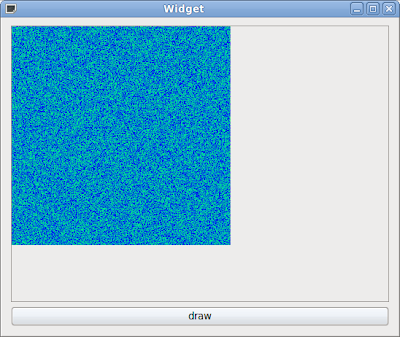# Qt : imagesc simple code

I am very interested with imagesc function in matlab. We can plot our data and plot image with user defined colormap. I have try to create a Qt: imagesc simple code using QPainter. This is a simple idea how to create Qt imagesc simple code. You can modified this code like your problem.

This code create a random data with size wdata(width) and hdata(height). I am create a winter colormap using colormap matlab function. You can get other colormap with looking at colormap matlab function. This is a code how to create winter colormap :

```//create winter colormap
int **drawingWidget::Winter()
{
int **cmap = array2int(m_colorMapLength, 4);
float *winter = new float[m_colorMapLength];
for (int i = 0; i < m_colorMapLength; i++)
{
winter[i] = 1.0f * i / (m_colorMapLength - 1);
cmap[i] = 255;
cmap[i] = 0;
cmap[i] = (int)(255 * winter[i]);
cmap[i] = (int)(255 * (1.0f - 0.5f * winter[i]));
}

delete [] winter;
return cmap;
}```

We can convert our data to colormap using this function :

```//convert our data to colormap color
QRgb drawingWidget::GetColor(float f)
{
int r, g, b, a;
float tmp1 = (m_colorMapLength * (f - minData) + (maxData - f));
float tmp2 = (maxData - minData);
int cindex = (int) round( tmp1/tmp2 );

if (cindex < 1)
cindex = 1;
if (cindex > m_colorMapLength)
cindex = m_colorMapLength;

//int alpha = cmap[cindex - 1, 0];
r = cmap[cindex - 1];
g = cmap[cindex - 1];
b = cmap[cindex - 1];
a = 255;
QRgb value = qRgba(r, g, b, a);

return value;
}```

You will get more information after reading my code. This is an output when running this Qt imagesc simple code :1.